CBSE Class 10 Sample Paper for 2021 Boards - Maths Standard

Class 10
Solutions of Sample Papers for Class 10 Boards

## A motorboat covers a distance of 16km upstream and 24km downstream in 6 hours. In the same time it covers a distance of 12 km upstream and 36km downstream. Find the speed of the boat in still water and that of the stream.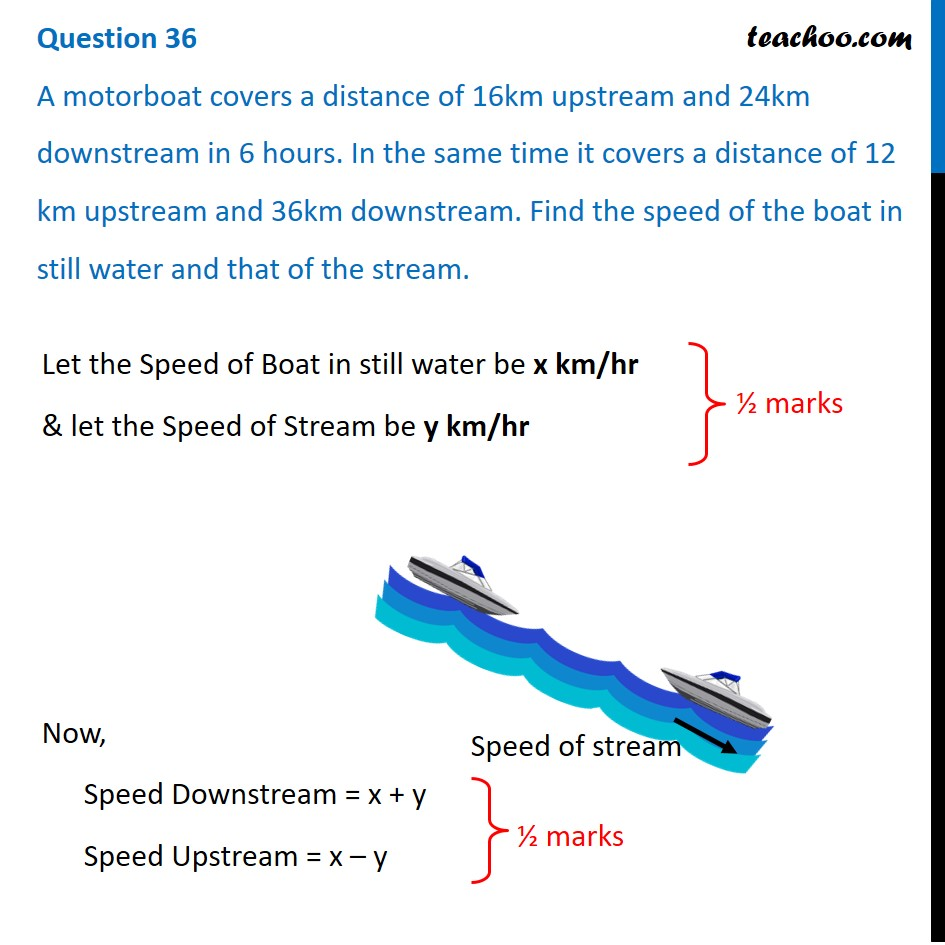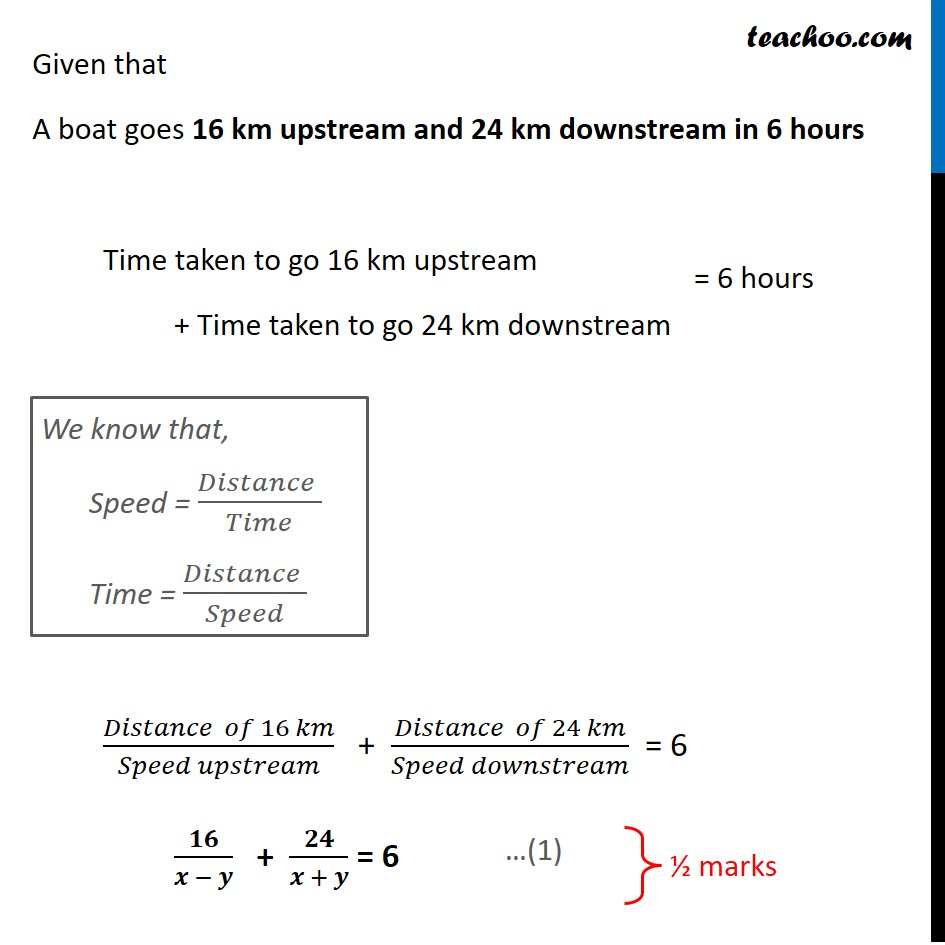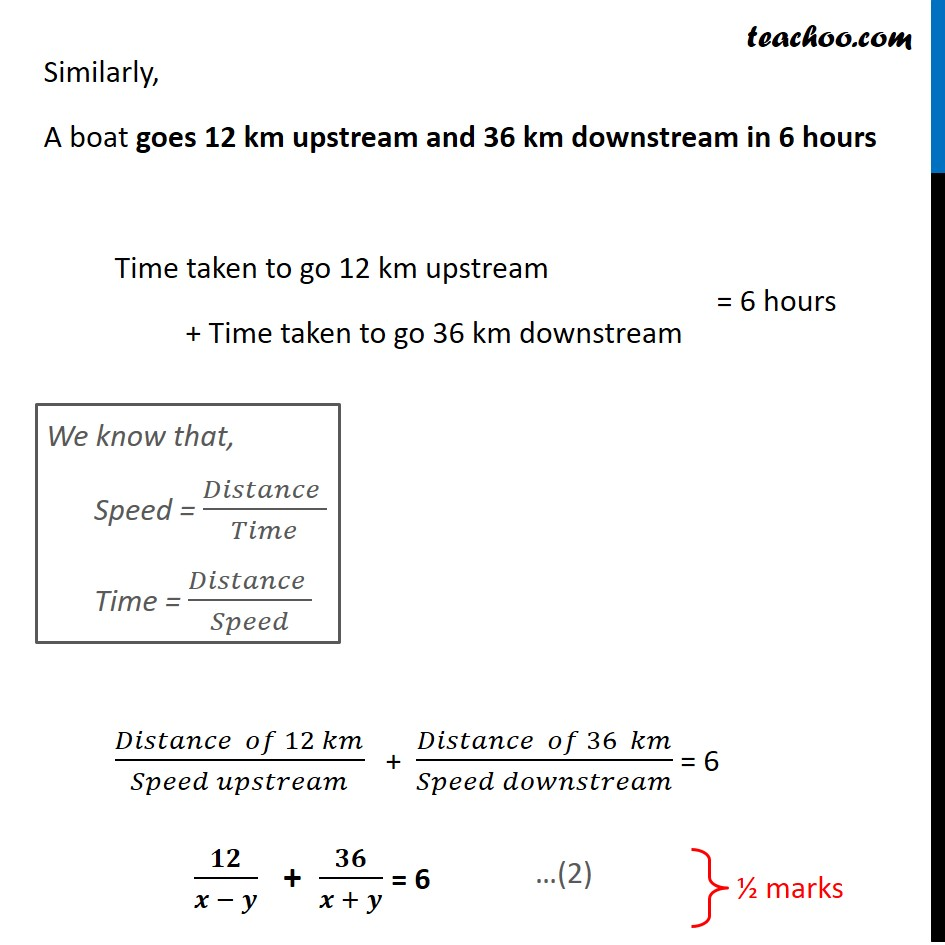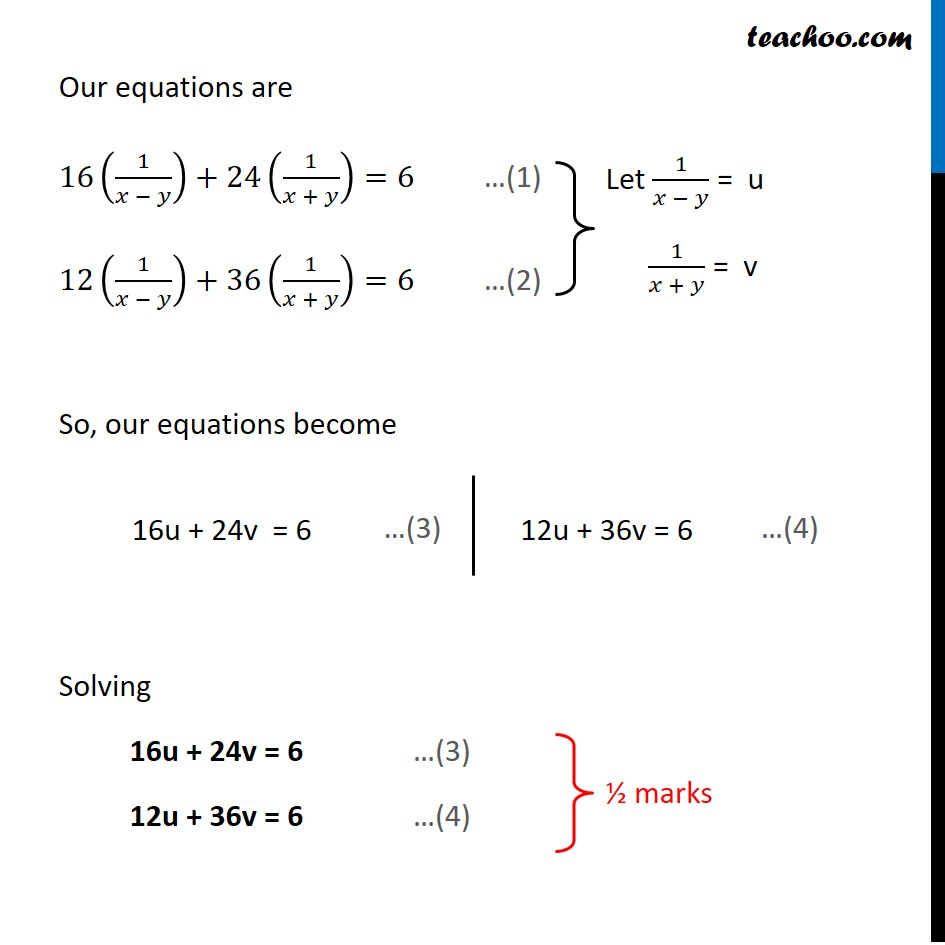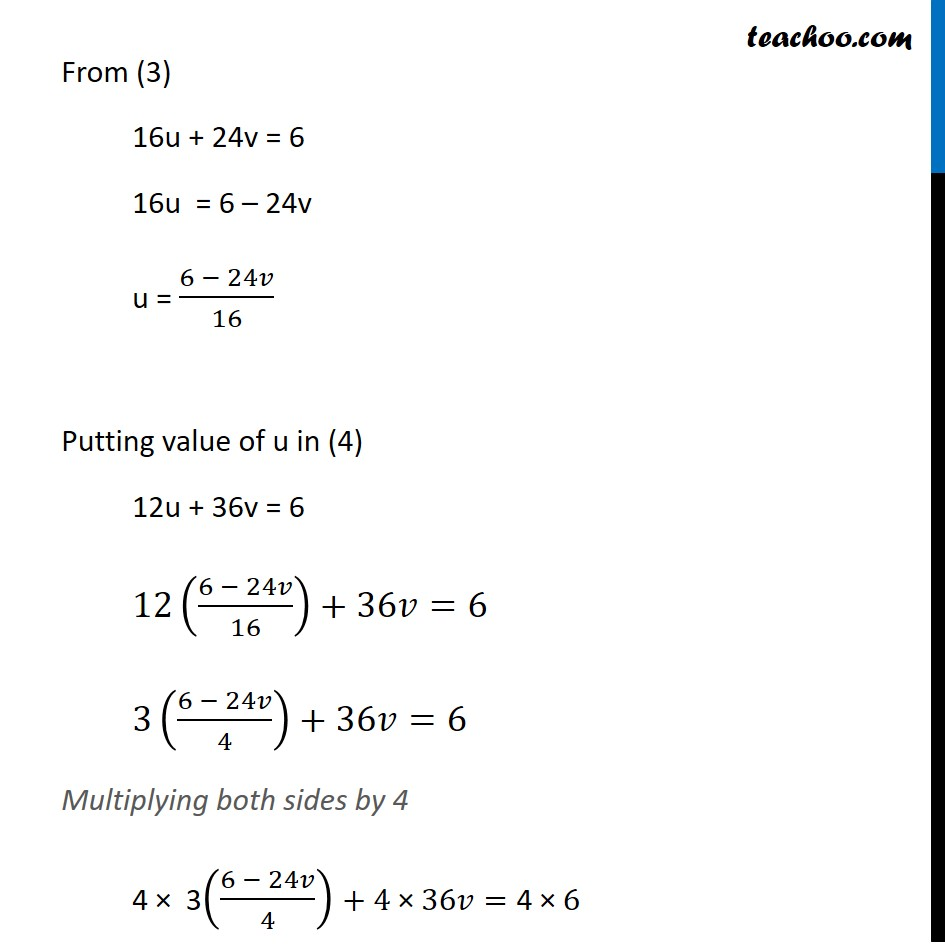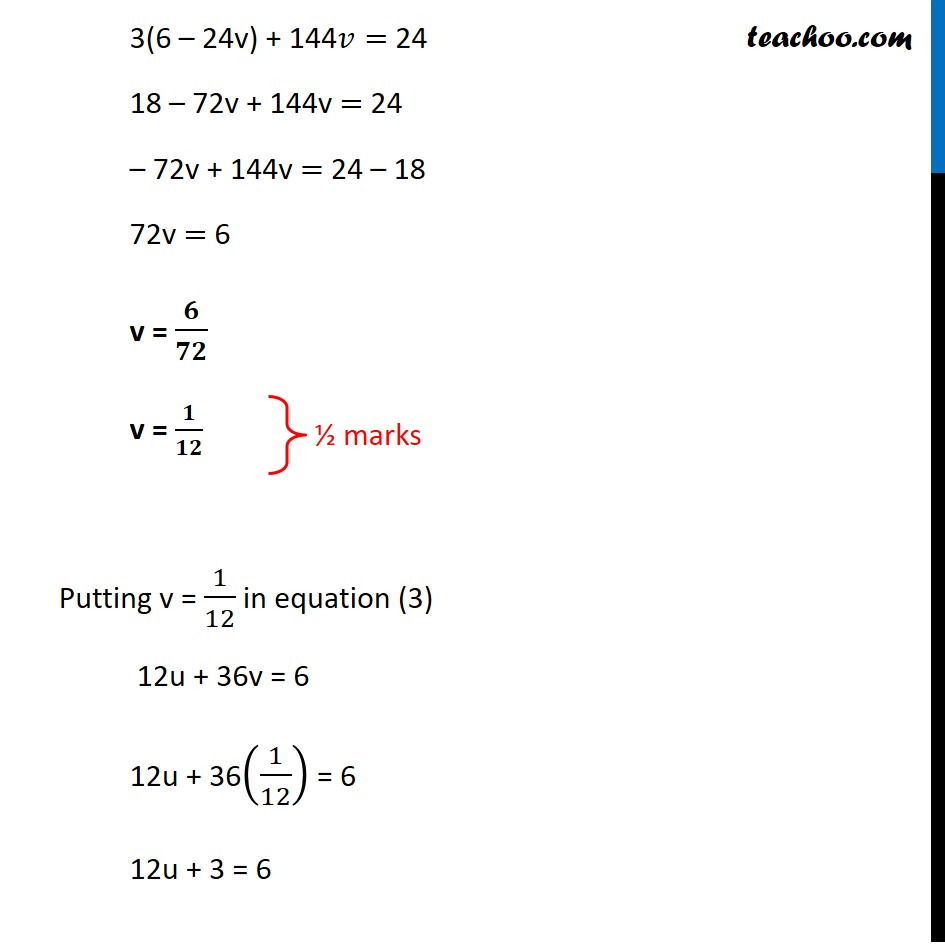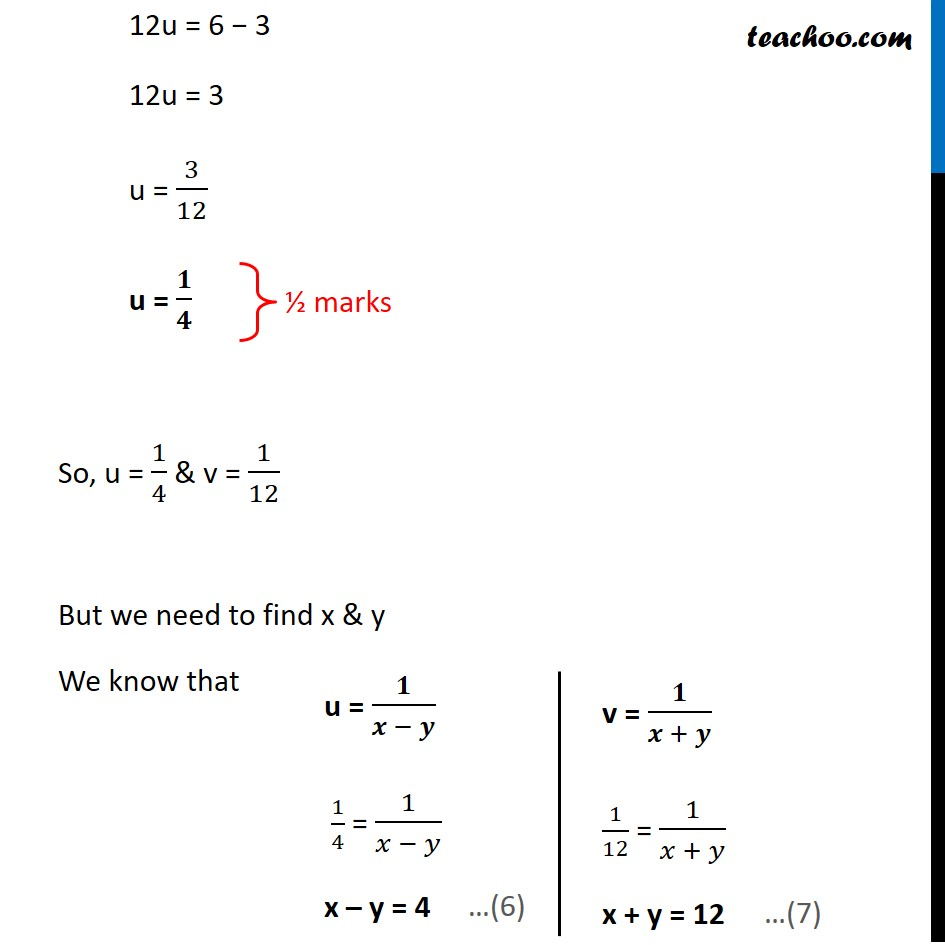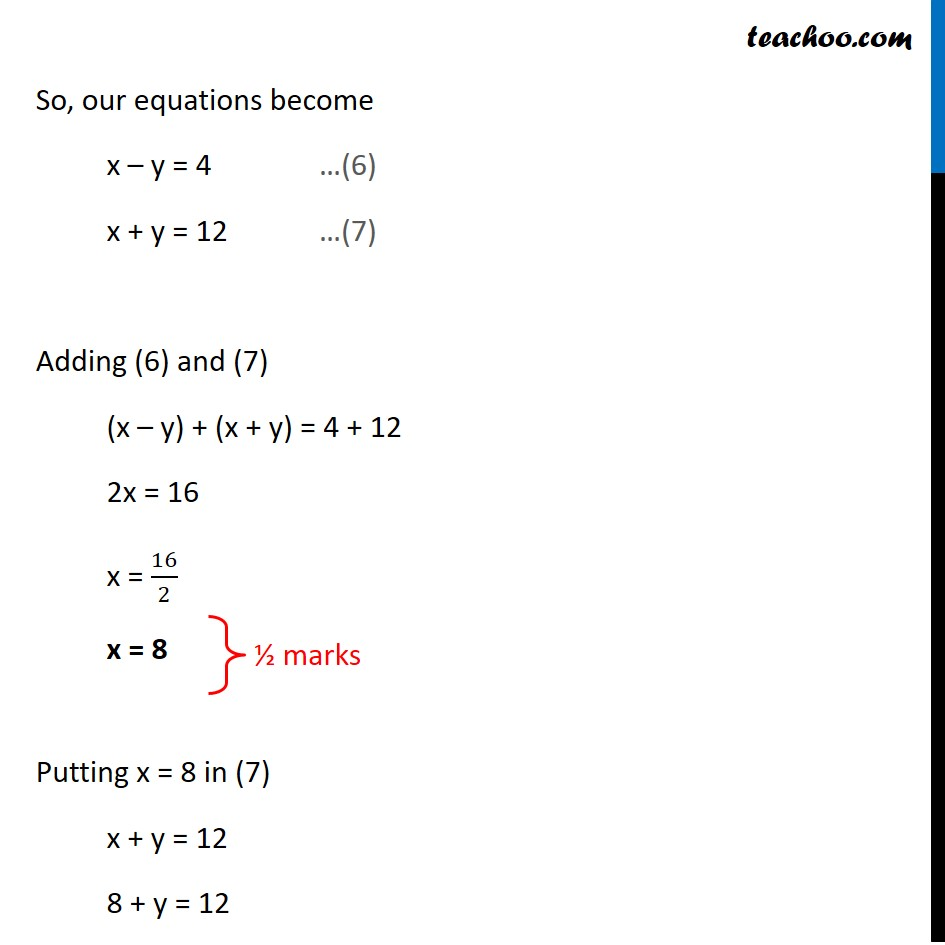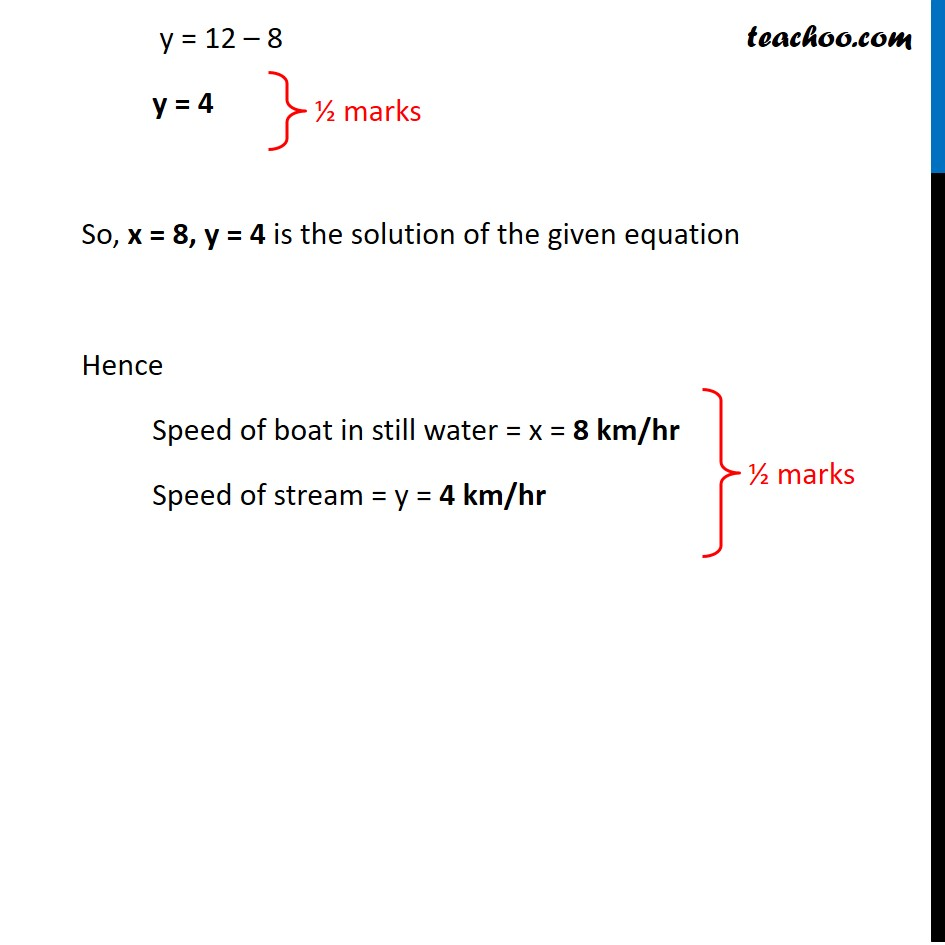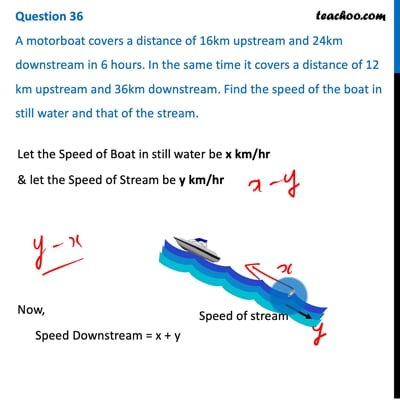This video is only available for Teachoo black users

Note : This is similar to Example 19 ,  Chapter 3 Class 10 Pair of Linear Equations in 2 Variables (NCERT Book)

Introducing your new favourite teacher - Teachoo Black, at only ₹83 per month

### Transcript

Question 36 A motorboat covers a distance of 16km upstream and 24km downstream in 6 hours. In the same time it covers a distance of 12 km upstream and 36km downstream. Find the speed of the boat in still water and that of the stream.Let the Speed of Boat in still water be x km/hr & let the Speed of Stream be y km/hr Now, Speed Downstream = x + y Speed Upstream = x – y Given that A boat goes 16 km upstream and 24 km downstream in 6 hours Time taken to go 16 km upstream + Time taken to go 24 km downstream (𝐷𝑖𝑠𝑡𝑎𝑛𝑐𝑒 𝑜𝑓 16 𝑘𝑚)/(𝑆𝑝𝑒𝑒𝑑 𝑢𝑝𝑠𝑡𝑟𝑒𝑎𝑚) + (𝐷𝑖𝑠𝑡𝑎𝑛𝑐𝑒 𝑜𝑓 24 𝑘𝑚)/(𝑆𝑝𝑒𝑒𝑑 𝑑𝑜𝑤𝑛𝑠𝑡𝑟𝑒𝑎𝑚) = 6 𝟏𝟔/(𝒙 − 𝒚) + 𝟐𝟒/(𝒙 + 𝒚) = 6 We know that, Speed = (𝐷𝑖𝑠𝑡𝑎𝑛𝑐𝑒 )/𝑇𝑖𝑚𝑒 Time = (𝐷𝑖𝑠𝑡𝑎𝑛𝑐𝑒 )/𝑆𝑝𝑒𝑒𝑑 Similarly, A boat goes 12 km upstream and 36 km downstream in 6 hours Time taken to go 12 km upstream + Time taken to go 36 km downstream (𝐷𝑖𝑠𝑡𝑎𝑛𝑐𝑒 𝑜𝑓 12 𝑘𝑚)/(𝑆𝑝𝑒𝑒𝑑 𝑢𝑝𝑠𝑡𝑟𝑒𝑎𝑚) + (𝐷𝑖𝑠𝑡𝑎𝑛𝑐𝑒 𝑜𝑓 36 𝑘𝑚)/(𝑆𝑝𝑒𝑒𝑑 𝑑𝑜𝑤𝑛𝑠𝑡𝑟𝑒𝑎𝑚) = 6 𝟏𝟐/(𝒙 − 𝒚) + 𝟑𝟔/(𝒙 + 𝒚) = 6 We know that, Speed = (𝐷𝑖𝑠𝑡𝑎𝑛𝑐𝑒 )/𝑇𝑖𝑚𝑒 Time = (𝐷𝑖𝑠𝑡𝑎𝑛𝑐𝑒 )/𝑆𝑝𝑒𝑒𝑑 Our equations are 16(1/(𝑥 − 𝑦))+24(1/(𝑥 + 𝑦))=6 …(1) 12(1/(𝑥 − 𝑦))+36(1/(𝑥 + 𝑦))=6 …(2) So, our equations become Solving 16u + 24v = 6 …(3) 12u + 36v = 6 …(4) Let 1/(𝑥 − 𝑦) = u 1/(𝑥 + 𝑦) = v 12u + 36v = 6 From (3) 16u + 24v = 6 16u = 6 – 24v u = (6 − 24𝑣)/16 Putting value of u in (4) 12u + 36v = 6 12((6 − 24𝑣)/16)+36𝑣=6 3((6 − 24𝑣)/4)+36𝑣=6 Multiplying both sides by 4 4 × 3((6 − 24𝑣)/4)+"4 ×" 36𝑣="4 ×" 6 3(6 – 24v) + 144𝑣= 24 18 – 72v + 144v = 24 – 72v + 144v = 24 – 18 72v = 6 v = 𝟔/𝟕𝟐 v = 𝟏/𝟏𝟐 Putting v = 1/12 in equation (3) 12u + 36v = 6 12u + 36(1/12) = 6 12u + 3 = 6 12u = 6 − 3 12u = 3 u = 3/12 u = 𝟏/𝟒 So, u = 1/4 & v = 1/12 But we need to find x & y We know that u = 𝟏/(𝒙 − 𝒚) 1/4 = 1/(𝑥 − 𝑦) x – y = 4 v = 𝟏/(𝒙 + 𝒚) 1/12 = 1/(𝑥 + 𝑦) x + y = 12 So, our equations become x – y = 4 …(6) x + y = 12 …(7) Adding (6) and (7) (x – y) + (x + y) = 4 + 12 2x = 16 x = 16/2 x = 8 Putting x = 8 in (7) x + y = 12 8 + y = 12 y = 12 – 8 y = 4 So, x = 8, y = 4 is the solution of the given equation Hence Speed of boat in still water = x = 8 km/hr Speed of stream = y = 4 km/hr y = 12 – 8 y = 4 So, x = 8, y = 4 is the solution of the given equation Hence Speed of boat in still water = x = 8 km/hr Speed of stream = y = 4 km/hr